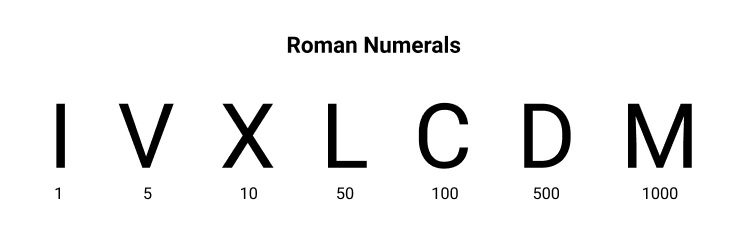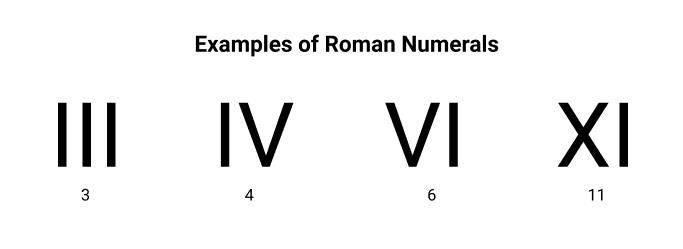×

Search anything:

# Integer to Roman Numeral Conversion

#### Algorithms List of Mathematical AlgorithmsFREE BOOK -> Problems for the day before your Coding Interview (on Amazon)

In this article, we have presented the algorithm to Convert Integer to Roman Numerals along with C++ implementation and step by step implementation.

## Table Content

1. Introduction
2. Algorithm
3. Code Development
4. Code Implementation
5. Final Considerations

Prerequisite: Mathematical Algorithms

## 1. Introduction

Roman numerals were developed in Ancient Rome to aid commerce at that time. A curiosity is that the number zero does not appear in this system, as the Romans of that time did not know it. The numbering system uses capital letters from the Latin alphabet, composed of only seven letters (Figure 1).Figure 1. Roman numbering system.

When these seven letters in different ways are combined, other numbers are formed (Figure 2).Figure 2. Combination of letters to form different numbers.

## 2. Algorithm

There are several ways to convert an integer to a Roman numeral.

One possible way to do it is using the following steps:

1. Choose an integer
2. Initially subtract this value with the highest possible value, 10 or 5.
3. After it is no longer possible to subtract by 10 or 5, subtract by 1. The subtraction is done until you get the value zero. See the example in the Table 1 below.

Table 1. Manual Conversion of Number 37 to Roman Numeral

#Interaction Integer Highest Decimal Value Highest Roman Numeral Preliminary Result
#1 37 10 X X
#2 27 10 X XX
#3 17 10 X XXX
#4 7 5 V XXXV
#5 2 1 I XXXVI
#6 1 1 I XXXVII

Therefore, 37 = XXXVII

These are the different Roman literals available:

``````1 -> I
2 -> II
3 -> III
4 -> IV (V means 5 and I infront means V - 1 = 5 - 1 = 4)
5 -> V
...
8 -> VIII
9 > IX
10 -> X
...
50 -> L
...
100 -> C
...
500 -> D
...
1000 -> M
``````

## 3. Code Development

For the conversion of an integer to a Roman numeral, a code in C++ was developed.

In the program header, the libraries were defined, and using namespace std; command to allows manipulating structures, classes, functions, constants, avoiding duplication of strings and other things (Figure 3).

``````#include <iostream>
#include <iomanip>
#include <cstring>
#include <stdlib.h>

using namespace std;
``````

In relation to the libraries:
<iostream>, handles the system's standard data stream (input and output);
<iomanip>, manages the presentation and processing of data streams;
<cstring>, manipulate the string;
<stdlib.h>, works as an operating system prompt emulator.

Inside the romanNumeral class, the characteristics of the variables are defined (Figure 4). The informed number by the user must be in the range from 1 to 3999 (Principle of Exclusion).

``````class romanNumerals {
public:
void result (char roman[]); // return Roman numeral
void integer (int number); // set the Integer number
void romanNum (char* roman); // set the Roman numeral
bool isError ();
int num;
};
``````

Figure 4. romanNumeral class is defining characteristics of variables and functions.

The next step was to define the error message and the chars characteristics (units, tens, hundreds, and thousands). The error message will appear if the entered number for the conversion is less than zero or greater/equal to 4000.

``````void romanNumerals::result(char roman[]){
int input1, input2=num;
roman=0;
char *thousands[]={ "M", "MM", "MMM"};
char *hundreds[]= { "C", "CC", "CCC", "CD", "D" ,"DC", "DCC", "DCCC", "CM"};
char *tens[]={ "X", "XX", "XXX", "XL", "L", "LX", "LXX", "LXXX", "XC"};
char *units[]={"I", "II", "III", "IV", "V", "VI", "VII", "VIII", "IX"};
int count;
if  ( num < 0 || num >=4000)// check if the number is equal to or less than 4000 and less than zero
{
cout<<endl;
cout<<"The number must be in the range of 0 to 3999."<<endl;
}
``````

Figure 5. Definition of char and error message.

In case the number entered by the user is greater than zero and less than 4000, it will be possible to convert the integer to a Roman numeral.

This block contains the calculations (units, tens, hundreds, and thousands) and the vectors to store the information (Figure 6).

``````else
{
for(count=0; count<4; count++)
{
if (input2 >= 1000  && input2 < 4000)  //returns the result in thousands
{
input1 = num/1000;
input2 = num%1000;
strcat ( roman, thousands[input1-1] );
}
if (input2 < 1000 && input2 >=100)  //returns the result in hundreds
{
input1 = (num%1000)/100;
input2 = num%100;
strcat ( roman, hundreds[input1-1] );
}
if (input2 < 100 && input2>= 10)  //returns the result in tens
{
input1 = ((num%1000)%100)/10;
input2 = num%10;
strcat ( roman, tens[input1-1] );
}
if (input2 <10 && input2 >= 1)    //returns the result in units
{
input1 = (((num%1000)%100)%10);
input2=0;
strcat ( roman, units[input1-1] );
}
}
cout<<endl;
cout<<"The Roman numeral is: "<<roman<<endl;
}
}
void romanNumerals::integer(int number)
{
num=number;
}
void romanNumerals::romanNum(char* roman)
{
int j=0;
num = 0;
while (roman[j] == 'M')
{
j++;
num+=1000;
}
j=0;
if (roman[j] == 'C' && roman[j+1] == 'M')
num+=900;
}
``````

Figure 6. Code block responsible for calculating and saving the information.

In the last block of code, the user must enter the number to convert to a Roman numeral. Checking will be done to confirm that it meets the specifications for the conversion (Figure 7).

``````int main()
{
romanNumerals check;
int number;
char romanInput;

cout<<"Enter the number: ";
cin>>number;
check.integer(number);
check.result(romanInput);
return 0;
}
``````

Figure 7. The last block of code, asking for input information for converting to Roman Numeral.

## 4. Code Implementation

With the code completed (Figure 8), some tests were performed (Table 2). The results obtained were as expected.

``````#include <iostream>
#include <iomanip>
#include <cstring>
#include <stdlib.h>
using namespace std;
class romanNumerals {
public:
void result (char roman[]);
void integer (int number);
void romanNum (char* roman);
bool isError ();
int num;
};
void romanNumerals::result(char roman[]){
int input1, input2=num;
roman=0;
char *thousands[]={ "M", "MM", "MMM"};
char *hundreds[]= { "C", "CC", "CCC", "CD", "D" ,"DC", "DCC", "DCCC", "CM"};
char *tens[]={ "X", "XX", "XXX", "XL", "L", "LX", "LXX", "LXXX", "XC"};
char *units[]={"I", "II", "III", "IV", "V", "VI", "VII", "VIII", "IX"};
int count;
if  ( num < 0 || num >=4000)// check if the number is equal to or less than 4000 and less than zero
{
cout<<endl;
cout<<"The number must be in the range of 0 to 3999."<<endl;
}
else{
for(count=0; count<4; count++){
if (input2 >= 1000  && input2 < 4000){
input1 = num/1000;
input2 = num%1000;
strcat ( roman, thousands[input1-1] );
}
if (input2 < 1000 && input2 >=100){
input1 = (num%1000)/100;
input2 = num%100;
strcat ( roman, hundreds[input1-1] );
}
if (input2 < 100 && input2>= 10)
{
input1 = ((num%1000)%100)/10;
input2 = num%10;
strcat ( roman, tens[input1-1] );
}
if (input2 <10 && input2 >= 1){
input1 = (((num%1000)%100)%10);
input2=0;
strcat ( roman, units[input1-1] );
}
}
cout<<endl;
cout<<"The Roman numeral is: "<<roman<<endl;
}
}
void romanNumerals::integer(int number){
num=number;
}
void romanNumerals::romanNum(char* roman){
int j=0;
num = 0;
while (roman[j] == 'M')
{
j++;
num+=1000;
}
j=0;
if (roman[j] == 'C' && roman[j+1] == 'M')
num+=900;
}
int main(){
romanNumerals check;
int number;
char romanInput;
cout<<"Enter the number: ";
cin>>number;
check.integer(number);
check.result(romanInput);
return 0;
}
``````

Figure 8. Complete Integer to Roman Numeral Conversion Code.

Table 2. Summary table of the results obtained in the conversion

Integer Roman numeral
7 VII
45 XLV
123 CXXIII
3999 MMMCMXCIX
4500 The number must be in the range of 0 to 3999.

## 5. Final Considerations

There are several types of code for converting integers to Roman numerals.
The development of this type of code brings benefits such as:

• helps to fix concepts;
• test different ways to solve the same problem;
• develops logical thinking contributing to the creation of versatile and cleaner codes;
• problem-solving faster and among many other things.

With this article at OpenGenus, you must have the complete idea of Converting Integer to Roman Numerals.#### Katia Kaori Kaminishikwahara

Software engineering student. I love tech and travel. I enjoy challenges and working out of my comfort zone.

Integer to Roman Numeral Conversion